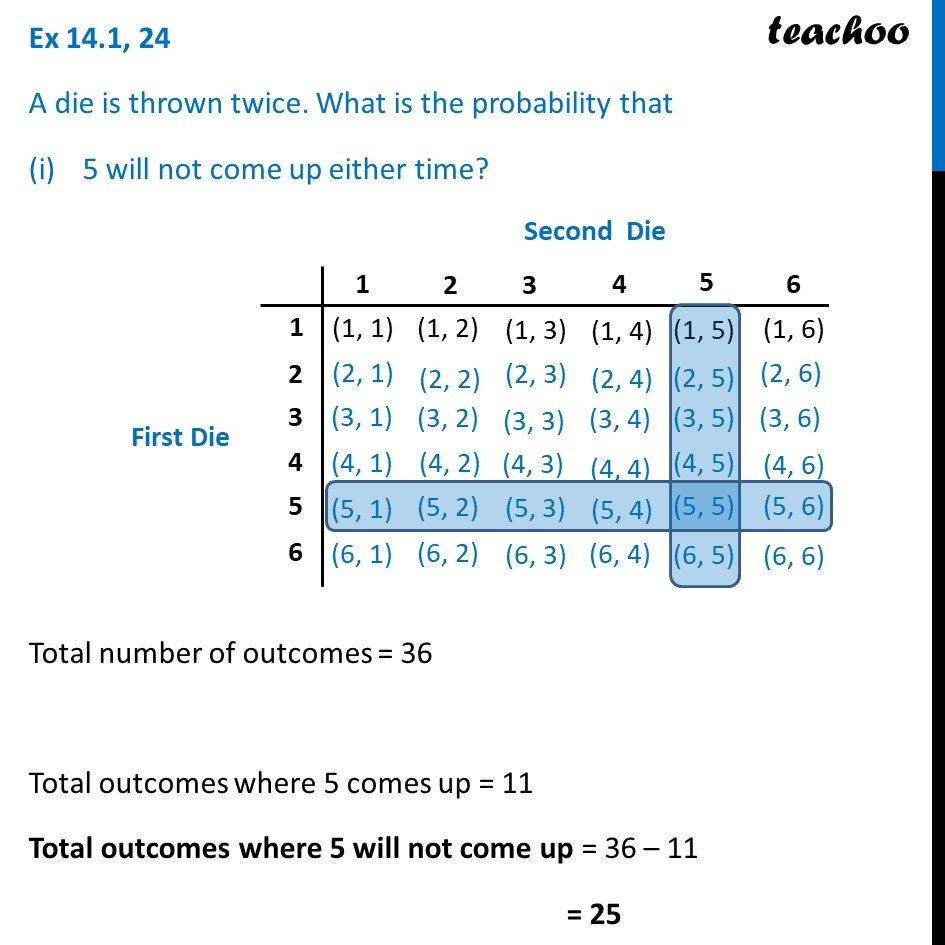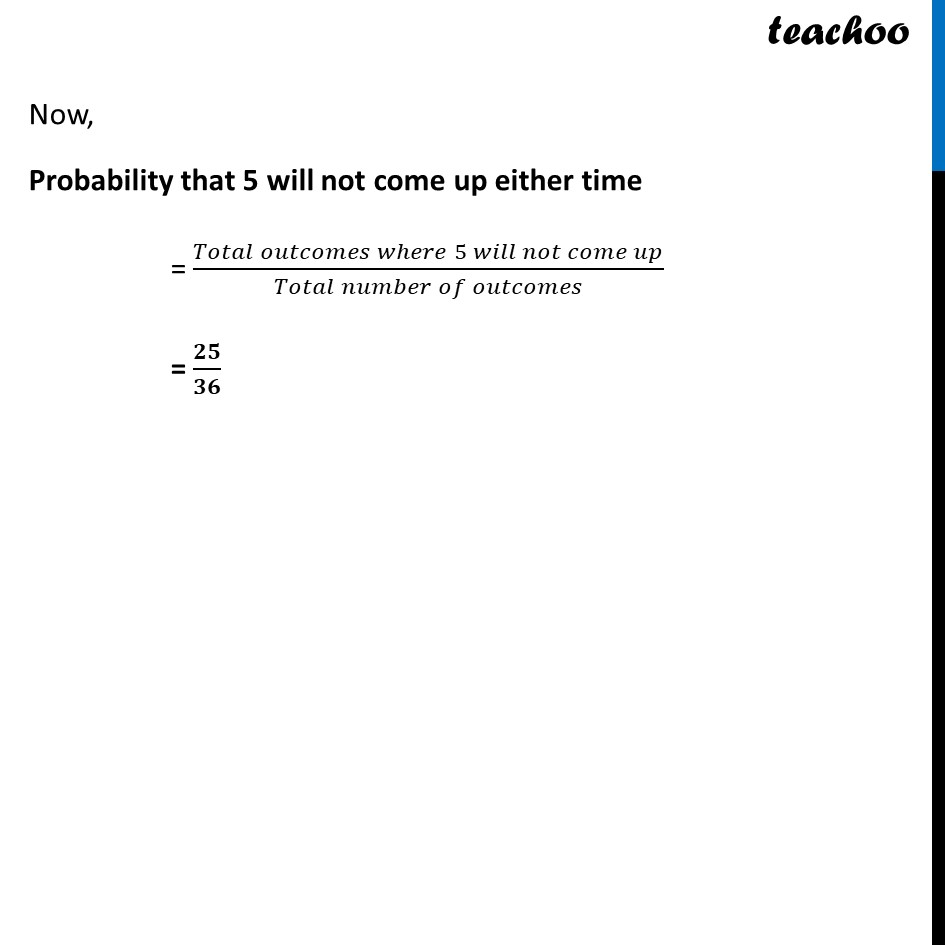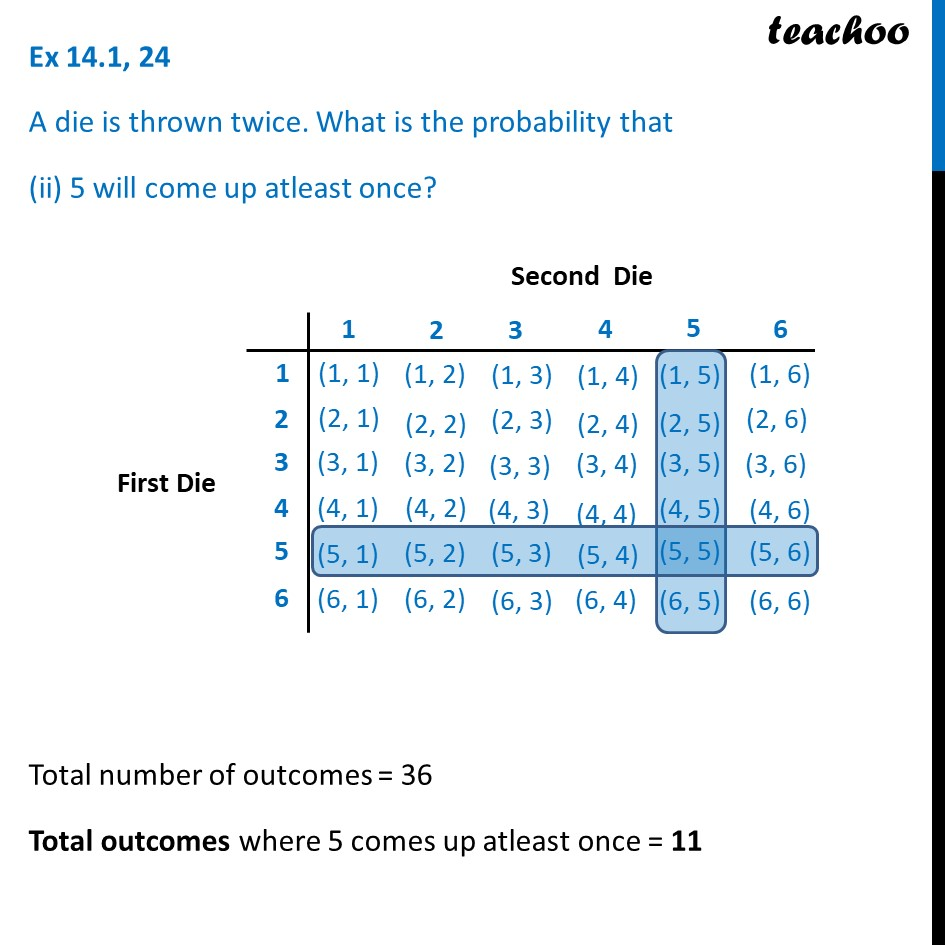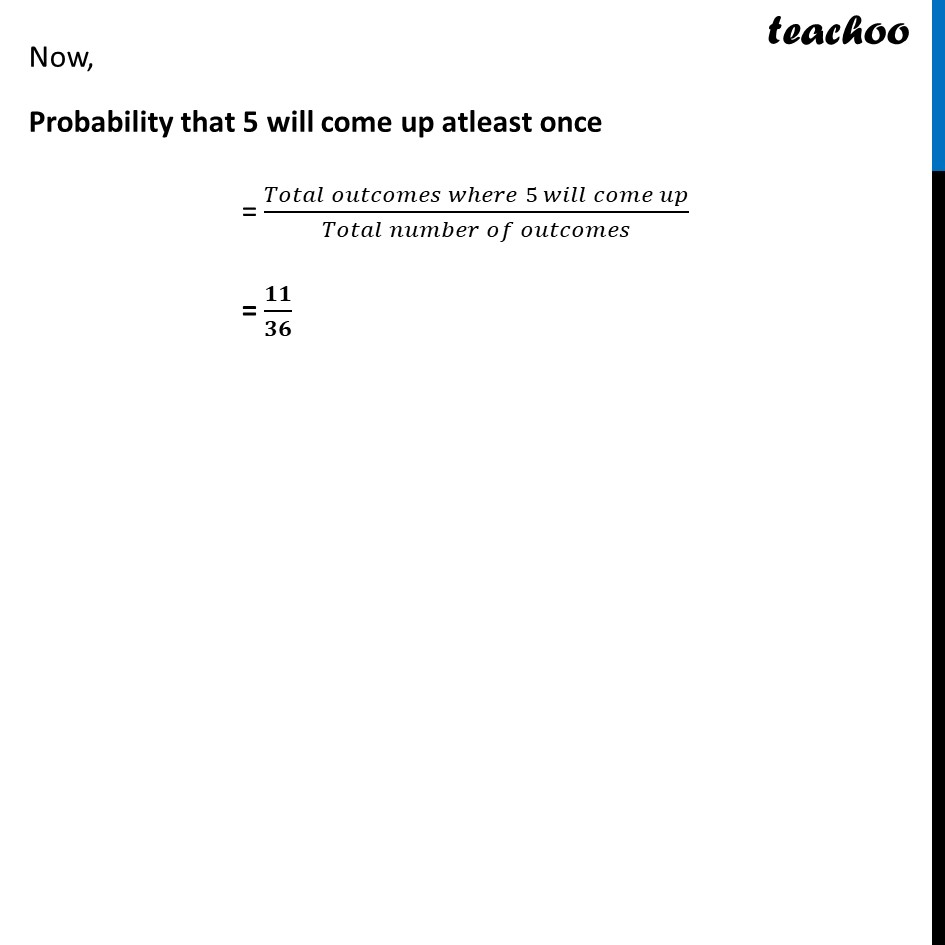Chapter 15 Class 10 Probability

Class 10
Important Questions for Exam - Class 10Learn in your speed, with individual attention - Teachoo Maths 1-on-1 Class

### Transcript

Ex 14.1, 24 A die is thrown twice. What is the probability that 5 will not come up either time? Total number of outcomes = 36 Total outcomes where 5 comes up = 11 Total outcomes where 5 will not come up = 36 – 11 = 25 Now, Probability that 5 will not come up either time = (𝑇𝑜𝑡𝑎𝑙 𝑜𝑢𝑡𝑐𝑜𝑚𝑒𝑠 𝑤ℎ𝑒𝑟𝑒 5 𝑤𝑖𝑙𝑙 𝑛𝑜𝑡 𝑐𝑜𝑚𝑒 𝑢𝑝)/(𝑇𝑜𝑡𝑎𝑙 𝑛𝑢𝑚𝑏𝑒𝑟 𝑜𝑓 𝑜𝑢𝑡𝑐𝑜𝑚𝑒𝑠) = 𝟐𝟓/𝟑𝟔 Ex 14.1, 24 A die is thrown twice. What is the probability that (ii) 5 will come up atleast once? Total number of outcomes = 36 Total outcomes where 5 comes up atleast once = 11 Now, Probability that 5 will come up atleast once = (𝑇𝑜𝑡𝑎𝑙 𝑜𝑢𝑡𝑐𝑜𝑚𝑒𝑠 𝑤ℎ𝑒𝑟𝑒 5 𝑤𝑖𝑙𝑙 𝑐𝑜𝑚𝑒 𝑢𝑝)/(𝑇𝑜𝑡𝑎𝑙 𝑛𝑢𝑚𝑏𝑒𝑟 𝑜𝑓 𝑜𝑢𝑡𝑐𝑜𝑚𝑒𝑠) = 𝟏𝟏/𝟑𝟔